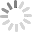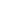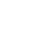## Free, outstanding support from WebAssist and your colleagues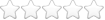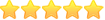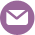# Calculate age from two dates

Thread began 6/26/2009 12:11 pm by Daryl | Last modified 6/26/2009 1:57 pm by Justin Nemeth | 5611 views | 2 replies |

##6/26/2009 12:11 pm  |  #1 DarylBeta Tester

### Calculate age from two dates

I would like to calculate an age of a person between two different date. The dates are both in yyyy-mm-dd

I am using the following;

`\$row_member_profile['dog_dob']\$row_member_profile['years']`

I have tried lots of different things but just can't get it to work.

Any help would be greatly appreciated.

##6/26/2009 1:37 pm  |  #2 DarylBeta Tester

I managed to solve it myself so thought I would post my solution for anyone else who might like to do this;

I created a file called datediff.php which contained the following;

php:
``<?php    function datediff(\$start_date,\$end_date,\$unit="y")        {            \$unit = strtoupper(\$unit);            \$start=strtotime(\$start_date);            if (\$start === -1) {                print("invalid start date");            }                        \$end=strtotime(\$end_date);                        if (\$end === -1) {                print("invalid end date");            }                        if (\$start > \$end) {                \$temp = \$start;                \$start = \$end;                \$end = \$temp;            }                        \$diff = \$end-\$start;                        \$day1 = date("j", \$start);            \$mon1 = date("n", \$start);            \$year1 = date("Y", \$start);            \$day2 = date("j", \$end);            \$mon2 = date("n", \$end);            \$year2 = date("Y", \$end);                        switch(\$unit) {                case "D":                    print(intval(\$diff/(24*60*60)));                    break;                case "M":                    if(\$day1>\$day2) {                        \$mdiff = ((\$year2-\$year1)*12)+(\$mon2-\$mon1-1);                    } else {                        \$mdiff = ((\$year2-\$year1)*12)+(\$mon2-\$mon1);                    }                    print(\$mdiff);                    break;                case "Y":                    if((\$mon1>\$mon2) || ((\$mon1==\$mon2) && (\$day1>\$day2))){                        \$ydiff = \$year2-\$year1-1;                    } else {                        \$ydiff = \$year2-\$year1;                    }                    print(\$ydiff);                    break;                case "YM":                    if(\$day1>\$day2) {                        if(\$mon1>=\$mon2) {                            \$ymdiff = 12+(\$mon2-\$mon1-1);                        } else {                            \$ymdiff = \$mon2-\$mon1-1;                        }                    } else {                        if(\$mon1>\$mon2) {                            \$ymdiff = 12+(\$mon2-\$mon1);                        } else {                            \$ymdiff = \$mon2-\$mon1;                        }                    }                    print(\$ymdiff);                    break;                case "YD":                    if((\$mon1>\$mon2) || ((\$mon1==\$mon2) &&(\$day1>\$day2))) {                        \$yddiff = intval((\$end - mktime(0, 0, 0, \$mon1, \$day1, \$year2-1))/(24*60*60));                                            } else {                        \$yddiff = intval((\$end - mktime(0, 0, 0, \$mon1, \$day1, \$year2))/(24*60*60));                    }                    print(\$yddiff);                    break;                case "MD":                    if(\$day1>\$day2) {                        \$mddiff = intval((\$end - mktime(0, 0, 0, \$mon2-1, \$day1, \$year2))/(24*60*60));                                            } else {                        \$mddiff = intval((\$end - mktime(0, 0, 0, \$mon2, \$day1, \$year2))/(24*60*60));                    }                    print(\$mddiff);                    break;                default:                 print("{Datedif Error: Unrecognized \\$unit parameter. Valid values are 'Y', 'M', 'D', 'YM'. Default is 'D'.}");                            }        }?>``

Then inlcuded it;

php:
``<?php include('dateDiff.php'); ?>``

Then added the code to my page;

php:
``<?php           \$date_1 = \$row_member_profile['dog_dob'];          \$date_2 = \$row_member_profile['years'];          datediff ("\$date_1","\$date_2","y"); ?>``

##6/26/2009 1:57 pm  |  #3 Justin Nemeth

If you are using MySQL (which I assume you are based on the \$row variable), check out the datediff function.

date-and-time-functions.html#function_datediff

That will give you the number of days between 2 dates. So you could do a statement like this to get the age.

`SELECT (DATEDIFF(dog_dob, years) / 365) AS age ....`

As for PHP, looks like your function will do what is needed, but it is probably overkill if you only want the years. Something like this would work. Basically determine the number of seconds between the dates, then divide by the number of seconds in a year.

php:
``<?php \$date_1 = \$row_member_profile['dog_dob'];\$date_2 = \$row_member_profile['years'];\$seconds = abs(strotime(\$date_1) - strotime(\$date_2));\$years = floor(\$seconds / (365 * 24 * 60 * 60));print \$years;?>``

I woud say the MySQL route is the easiest to do.

## Build websites with a little help from your friends

Your friends over here at WebAssist! These Dreamweaver extensions will assist you in building unlimited, custom websites.

## Build websites from already-built web applications

These out-of-the-box solutions provide you proven, tested applications that can be up and running now.  Build a store, a gallery, or a web-based email solution.

## Everything else!

Close Window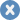Account or customer service questions?

Need technical support?

ContentLayoutEase of use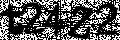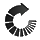We do not respond to comments submitted from this page directly, but we do read and analyze any feedback and will use it to help make your experience better in the future.

Close Window## We were unable to retrieve the attached file

Close Window### Attach and remove files

• Text Formatting
• Insert Element
• Block Formatting
• Cancel
Close Window#### Enter the URL you would like to link to in your post

Close Window#### This is how you use right click RTF editing

Enable right click RTF editing option allows you to add html markup into your tutorial such as images, bulleted lists, files and more...-- click to close --